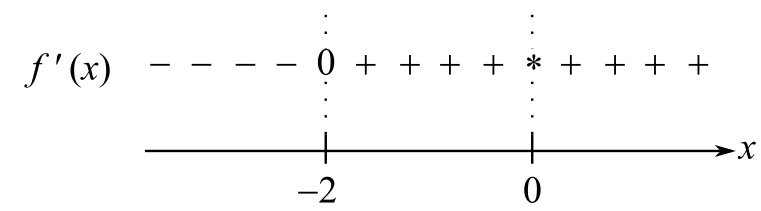Chapter 10.5, Problem 2CP### Mathematical Applications for the ...

11th Edition
Ronald J. Harshbarger + 1 other
ISBN: 9781305108042

#### Solutions

Chapter
Section### Mathematical Applications for the ...

11th Edition
Ronald J. Harshbarger + 1 other
ISBN: 9781305108042
Textbook Problem

# Let f ( x ) = x 3 − 16 x + 1 ; then  f ' ( x ) = 2 x 3 + 16 x 2  and  f " ( x ) = 2 x 3 − 32 x 3 .Use these to determine whether the following are true or false.(a) There are no asymptotes.(b) f ' ( x ) = 0  when  x =-2 (c) A partial sign diagram for f ' ( x ) is*means that f ' ( 0 ) is undefined.(d) There is a relative minimum at x = − 2 .(e) A partial sign diagram for f " ( x ) is*means that/"(0) is undefined.(f) There are points of inflection at x = 0 and x = 16 3 .

(a)

To determine

Whether the provided statement “f(x)=x316x+1 has no asymptotes.” is true or false.

Explanation

Consider the provided function,

f(x)=2x+10x1

Now, it is a rational function and the vertical asymptote of a rational function y=f(x)g(x) exists at x=c where g

(b)

To determine

Whether the provided statement “f(x)=0 when x=2 if f(x)=2x3+16x2.” is true or false.

(c)

To determine

Whether the provided statement is true or false “A partial sign diagram for f(x) isif f(x)=x316x+1 and f(x)=2x3+16x2

(d)

To determine

Whether the provided statement “There is a relative minimum at x=2 if f(x)=2x3+16x2” is true or false.

(e)

To determine

Whether the provided statement “A partial sign diagram for f(x) isif f(x)=2x332x3.” is true or false.

(f)

To determine

Whether the provided statement “There are points of inflection at x=163 and x=0 if f(x)=2x332x3.” is true or false.

### Still sussing out bartleby?

Check out a sample textbook solution.

See a sample solution

#### The Solution to Your Study Problems

Bartleby provides explanations to thousands of textbook problems written by our experts, many with advanced degrees!

Get Started

#### Solve the equations in Exercises 126. 2x3x2x3x+1=0

Finite Mathematics and Applied Calculus (MindTap Course List)

#### In Exercises 19-24, find the functions f + g, f g, fg, and fg. 19. f(x) = x2 + 5; g(x) = x2

Applied Calculus for the Managerial, Life, and Social Sciences: A Brief Approach

#### True or False: f(x) = 4x2 8x + 1 is increasing on (0, 1).

Study Guide for Stewart's Single Variable Calculus: Early Transcendentals, 8th